# Mean State

Period Mean (original grids) [K]
Model Period Mean (intersection) [K]
Model Period Mean (complement) [K]
Benchmark Period Mean (intersection) [K]
Benchmark Period Mean (complement) [K]
Bias [K]
RMSE [K]
Phase Shift [months]
Bias Score 
RMSE Score 
Seasonal Cycle Score 
Spatial Distribution Score 
Interannual Variability Score 
Overall Score 
Benchmark [-] 299.
CRUNCEPv7 [-] 299. 299. 301. 298. 300. 0.167 0.738 0.323 0.62 0.79 0.96 0.98 0.96 0.85
GSWP3v1 [-] 299. 299. 300. 298. 300. 0.285 0.690 0.366 0.67 0.78 0.97 1.0 0.96 0.86
WATCH [-] 298. 298. 301. 298. 300. 0.0939 1.05 0.579 0.61 0.53 0.94 1.0 0.91 0.75
Period Mean (original grids) [K]
Model Period Mean (intersection) [K]
Model Period Mean (complement) [K]
Benchmark Period Mean (intersection) [K]
Benchmark Period Mean (complement) [K]
Bias [K]
RMSE [K]
Phase Shift [months]
Bias Score 
RMSE Score 
Seasonal Cycle Score 
Spatial Distribution Score 
Interannual Variability Score 
Overall Score 
Benchmark [-] 300.
CRUNCEPv7 [-] 301. 301. 301. 300. 300. 0.521 0.852 0.195 0.73 0.85 0.98 1.0 0.97 0.90
GSWP3v1 [-] 301. 301. 301. 300. 300. 0.473 0.802 0.168 0.75 0.86 0.99 0.99 0.96 0.90
WATCH [-] 300. 300. 301. 300. 300. -0.210 1.26 0.319 0.73 0.67 0.98 1.0 0.91 0.83
Period Mean (original grids) [K]
Model Period Mean (intersection) [K]
Model Period Mean (complement) [K]
Benchmark Period Mean (intersection) [K]
Benchmark Period Mean (complement) [K]
Bias [K]
RMSE [K]
Phase Shift [months]
Bias Score 
RMSE Score 
Seasonal Cycle Score 
Spatial Distribution Score 
Interannual Variability Score 
Overall Score 
Benchmark [-] 295.
CRUNCEPv7 [-] 296. 295. 297. 295. 297. 0.445 0.865 0.404 0.71 0.87 0.95 1.0 0.95 0.89
GSWP3v1 [-] 296. 296. 296. 295. 297. 0.505 0.854 0.273 0.71 0.87 0.97 1.0 0.96 0.89
WATCH [-] 295. 295. 296. 295. 297. -0.155 1.25 0.594 0.69 0.70 0.92 0.99 0.85 0.81
Period Mean (original grids) [K]
Model Period Mean (intersection) [K]
Model Period Mean (complement) [K]
Benchmark Period Mean (intersection) [K]
Benchmark Period Mean (complement) [K]
Bias [K]
RMSE [K]
Phase Shift [months]
Bias Score 
RMSE Score 
Seasonal Cycle Score 
Spatial Distribution Score 
Interannual Variability Score 
Overall Score 
Benchmark [-] 262.
CRUNCEPv7 [-] 260. 260. 263. 262. 266. -1.00 1.60 0.0159 0.92 0.94 1.0 1.0 0.92 0.95
GSWP3v1 [-] 262. 261. 267. 262. 266. 0.258 1.13 0.0161 0.94 0.96 1.0 1.0 0.94 0.97
WATCH [-] 260. 260. 263. 261. 265. -1.08 1.99 0.00912 0.92 0.91 1.0 1.0 0.88 0.94
Period Mean (original grids) [K]
Model Period Mean (intersection) [K]
Model Period Mean (complement) [K]
Benchmark Period Mean (intersection) [K]
Benchmark Period Mean (complement) [K]
Bias [K]
RMSE [K]
Phase Shift [months]
Bias Score 
RMSE Score 
Seasonal Cycle Score 
Spatial Distribution Score 
Interannual Variability Score 
Overall Score 
Benchmark [-] 299.
CRUNCEPv7 [-] 299. 299. 300. 299. 299. -0.0330 0.793 0.616 0.42 0.68 0.91 1.0 0.88 0.76
GSWP3v1 [-] 299. 299. 300. 299. 299. 0.226 0.750 0.486 0.42 0.72 0.93 0.96 0.91 0.78
WATCH [-] 299. 299. 300. 299. 299. 0.0793 0.999 1.01 0.40 0.46 0.85 1.0 0.80 0.66
Period Mean (original grids) [K]
Model Period Mean (intersection) [K]
Model Period Mean (complement) [K]
Benchmark Period Mean (intersection) [K]
Benchmark Period Mean (complement) [K]
Bias [K]
RMSE [K]
Phase Shift [months]
Bias Score 
RMSE Score 
Seasonal Cycle Score 
Spatial Distribution Score 
Interannual Variability Score 
Overall Score 
Benchmark [-] 271.
CRUNCEPv7 [-] 271. 270. 274. 271. 274. -0.737 1.06 0.00890 0.94 0.96 1.0 1.0 0.98 0.97
GSWP3v1 [-] 271. 271. 275. 271. 274. -0.0249 0.647 0.00842 0.97 0.97 1.0 1.0 0.98 0.98
WATCH [-] 270. 270. 273. 271. 274. -0.842 1.44 0.00791 0.93 0.93 1.0 1.0 0.95 0.96
Period Mean (original grids) [K]
Model Period Mean (intersection) [K]
Model Period Mean (complement) [K]
Benchmark Period Mean (intersection) [K]
Benchmark Period Mean (complement) [K]
Bias [K]
RMSE [K]
Phase Shift [months]
Bias Score 
RMSE Score 
Seasonal Cycle Score 
Spatial Distribution Score 
Interannual Variability Score 
Overall Score 
Benchmark [-] 280.
CRUNCEPv7 [-] 280. 280. 287. 280. 285. -0.0730 1.17 0.0271 0.90 0.95 1.0 1.0 0.96 0.96
GSWP3v1 [-] 281. 280. 288. 280. 285. 0.249 1.09 0.0222 0.90 0.96 1.0 1.0 0.96 0.96
WATCH [-] 280. 279. 287. 280. 285. -0.454 1.59 0.0272 0.88 0.91 1.0 1.0 0.92 0.94
Period Mean (original grids) [K]
Model Period Mean (intersection) [K]
Model Period Mean (complement) [K]
Benchmark Period Mean (intersection) [K]
Benchmark Period Mean (complement) [K]
Bias [K]
RMSE [K]
Phase Shift [months]
Bias Score 
RMSE Score 
Seasonal Cycle Score 
Spatial Distribution Score 
Interannual Variability Score 
Overall Score 
Benchmark [-] 297.
CRUNCEPv7 [-] 297. 297. 300. 297. 300. 0.195 1.12 0.170 0.75 0.89 0.98 1.0 0.94 0.91
GSWP3v1 [-] 297. 297. 300. 297. 300. 0.110 1.08 0.181 0.77 0.88 0.98 1.0 0.95 0.91
WATCH [-] 297. 297. 300. 297. 300. 0.147 1.46 0.229 0.75 0.79 0.98 1.0 0.90 0.87
Period Mean (original grids) [K]
Model Period Mean (intersection) [K]
Model Period Mean (complement) [K]
Benchmark Period Mean (intersection) [K]
Benchmark Period Mean (complement) [K]
Bias [K]
RMSE [K]
Phase Shift [months]
Bias Score 
RMSE Score 
Seasonal Cycle Score 
Spatial Distribution Score 
Interannual Variability Score 
Overall Score 
Benchmark [-] 264.
CRUNCEPv7 [-] 263. 263. 264. 264. 264. -1.09 1.43 0.0193 0.93 0.95 1.0 1.0 0.97 0.97
GSWP3v1 [-] 264. 264. 267. 264. 264. 0.0517 0.717 0.0135 0.97 0.97 1.0 1.0 0.96 0.98
WATCH [-] 263. 263. 264. 264. 264. -0.917 1.62 0.0141 0.94 0.92 1.0 1.0 0.95 0.95
Period Mean (original grids) [K]
Model Period Mean (intersection) [K]
Model Period Mean (complement) [K]
Benchmark Period Mean (intersection) [K]
Benchmark Period Mean (complement) [K]
Bias [K]
RMSE [K]
Phase Shift [months]
Bias Score 
RMSE Score 
Seasonal Cycle Score 
Spatial Distribution Score 
Interannual Variability Score 
Overall Score 
Benchmark [-] 295.
CRUNCEPv7 [-] 295. 295. 293. 295. 294. 0.323 0.624 0.0892 0.89 0.93 0.99 1.0 0.95 0.95
GSWP3v1 [-] 295. 295. 294. 295. 294. 0.479 0.699 0.0807 0.88 0.93 0.99 1.0 0.97 0.95
WATCH [-] 295. 295. 293. 295. 294. 0.264 0.830 0.116 0.88 0.89 0.99 1.0 0.95 0.93
Period Mean (original grids) [K]
Model Period Mean (intersection) [K]
Model Period Mean (complement) [K]
Benchmark Period Mean (intersection) [K]
Benchmark Period Mean (complement) [K]
Bias [K]
RMSE [K]
Phase Shift [months]
Bias Score 
RMSE Score 
Seasonal Cycle Score 
Spatial Distribution Score 
Interannual Variability Score 
Overall Score 
Benchmark [-] 282.
CRUNCEPv7 [-] 281. 281. 284. 282. 286. -0.172 0.983 0.147 0.89 0.92 0.99 1.0 0.95 0.95
GSWP3v1 [-] 282. 282. 284. 282. 286. 0.117 0.866 0.145 0.90 0.94 0.99 1.0 0.97 0.96
WATCH [-] 281. 281. 283. 282. 285. -0.309 1.21 0.234 0.88 0.90 0.98 1.0 0.92 0.93
Period Mean (original grids) [K]
Model Period Mean (intersection) [K]
Model Period Mean (complement) [K]
Benchmark Period Mean (intersection) [K]
Benchmark Period Mean (complement) [K]
Bias [K]
RMSE [K]
Phase Shift [months]
Bias Score 
RMSE Score 
Seasonal Cycle Score 
Spatial Distribution Score 
Interannual Variability Score 
Overall Score 
Benchmark [-] 287.
CRUNCEPv7 [-] 282. 286. 244. 287. 292. -0.107 1.02 0.157 0.82 0.90 0.98 1.0 0.95 0.93
GSWP3v1 [-] 283. 287. 249. 287. 292. 0.227 0.898 0.137 0.83 0.91 0.99 1.0 0.96 0.93
WATCH [-] 282. 286. 248. 286. 292. -0.345 1.37 0.248 0.80 0.81 0.97 1.0 0.91 0.88
Period Mean (original grids) [K]
Model Period Mean (intersection) [K]
Model Period Mean (complement) [K]
Benchmark Period Mean (intersection) [K]
Benchmark Period Mean (complement) [K]
Bias [K]
RMSE [K]
Phase Shift [months]
Bias Score 
RMSE Score 
Seasonal Cycle Score 
Spatial Distribution Score 
Interannual Variability Score 
Overall Score 
Benchmark [-] 296.
CRUNCEPv7 [-] 296. 296. 297. 296. 297. 0.240 0.867 0.138 0.89 0.94 0.99 1.0 0.96 0.95
GSWP3v1 [-] 296. 296. 296. 296. 297. 0.191 0.824 0.134 0.90 0.94 0.99 1.0 0.96 0.95
WATCH [-] 295. 295. 296. 296. 297. -0.455 1.37 0.239 0.86 0.88 0.98 1.0 0.91 0.92
Period Mean (original grids) [K]
Model Period Mean (intersection) [K]
Model Period Mean (complement) [K]
Benchmark Period Mean (intersection) [K]
Benchmark Period Mean (complement) [K]
Bias [K]
RMSE [K]
Phase Shift [months]
Bias Score 
RMSE Score 
Seasonal Cycle Score 
Spatial Distribution Score 
Interannual Variability Score 
Overall Score 
Benchmark [-] 265.
CRUNCEPv7 [-] 264. 264. 261. 265. 266. -0.989 1.43 0.0106 0.92 0.93 1.0 1.0 0.94 0.96
GSWP3v1 [-] 265. 265. 266. 265. 266. 0.255 0.978 0.0188 0.94 0.95 1.0 1.0 0.96 0.97
WATCH [-] 264. 264. 262. 265. 266. -0.618 1.32 0.0138 0.94 0.93 1.0 1.0 0.95 0.96
Period Mean (original grids) [K]
Model Period Mean (intersection) [K]
Model Period Mean (complement) [K]
Benchmark Period Mean (intersection) [K]
Benchmark Period Mean (complement) [K]
Bias [K]
RMSE [K]
Phase Shift [months]
Bias Score 
RMSE Score 
Seasonal Cycle Score 
Spatial Distribution Score 
Interannual Variability Score 
Overall Score 
Benchmark [-] 296.
CRUNCEPv7 [-] 295. 295. 298. 296. 298. 0.206 1.20 0.263 0.67 0.85 0.98 1.0 0.93 0.88
GSWP3v1 [-] 296. 295. 298. 296. 298. 0.401 1.19 0.241 0.67 0.86 0.98 1.0 0.94 0.89
WATCH [-] 295. 295. 298. 296. 298. 0.241 1.56 0.280 0.64 0.74 0.98 1.0 0.87 0.83
Period Mean (original grids) [K]
Model Period Mean (intersection) [K]
Model Period Mean (complement) [K]
Benchmark Period Mean (intersection) [K]
Benchmark Period Mean (complement) [K]
Bias [K]
RMSE [K]
Phase Shift [months]
Bias Score 
RMSE Score 
Seasonal Cycle Score 
Spatial Distribution Score 
Interannual Variability Score 
Overall Score 
Benchmark [-] 286.
CRUNCEPv7 [-] 286. 286. 294. 286. 292. -0.0907 0.670 0.0345 0.95 0.95 1.0 1.0 0.97 0.97
GSWP3v1 [-] 286. 286. 294. 286. 292. 0.281 0.608 0.0307 0.94 0.96 1.0 1.0 0.97 0.97
WATCH [-] 285. 285. 293. 286. 292. -0.648 1.05 0.0379 0.92 0.92 1.0 1.0 0.95 0.95
Period Mean (original grids) [K]
Model Period Mean (intersection) [K]
Model Period Mean (complement) [K]
Benchmark Period Mean (intersection) [K]
Benchmark Period Mean (complement) [K]
Bias [K]
RMSE [K]
Phase Shift [months]
Bias Score 
RMSE Score 
Seasonal Cycle Score 
Spatial Distribution Score 
Interannual Variability Score 
Overall Score 
Benchmark [-] 275.
CRUNCEPv7 [-] 274. 274. 277. 274. 278. -0.630 1.01 0.0424 0.92 0.95 1.0 1.0 0.97 0.96
GSWP3v1 [-] 274. 274. 278. 274. 278. 0.109 0.688 0.0418 0.94 0.96 1.0 1.0 0.97 0.97
WATCH [-] 273. 273. 277. 274. 278. -0.691 1.28 0.0598 0.92 0.92 1.0 1.0 0.95 0.95
Period Mean (original grids) [K]
Model Period Mean (intersection) [K]
Model Period Mean (complement) [K]
Benchmark Period Mean (intersection) [K]
Benchmark Period Mean (complement) [K]
Bias [K]
RMSE [K]
Phase Shift [months]
Bias Score 
RMSE Score 
Seasonal Cycle Score 
Spatial Distribution Score 
Interannual Variability Score 
Overall Score 
Benchmark [-] 284.
CRUNCEPv7 [-] 283. 283. 287. 284. 286. -0.0645 1.18 0.0249 0.88 0.92 1.0 1.0 0.97 0.95
GSWP3v1 [-] 284. 284. 287. 284. 286. 0.371 1.11 0.0268 0.88 0.94 1.0 1.0 0.96 0.95
WATCH [-] 283. 283. 287. 283. 286. -0.219 1.67 0.101 0.86 0.88 0.99 1.0 0.92 0.92
Period Mean (original grids) [K]
Model Period Mean (intersection) [K]
Model Period Mean (complement) [K]
Benchmark Period Mean (intersection) [K]
Benchmark Period Mean (complement) [K]
Bias [K]
RMSE [K]
Phase Shift [months]
Bias Score 
RMSE Score 
Seasonal Cycle Score 
Spatial Distribution Score 
Interannual Variability Score 
Overall Score 
Benchmark [-] 299.
CRUNCEPv7 [-] 299. 299. 300. 299. 299. -0.0597 0.717 0.460 0.65 0.76 0.94 1.0 0.94 0.84
GSWP3v1 [-] 299. 299. 300. 299. 299. 0.168 0.688 0.391 0.65 0.79 0.95 1.0 0.93 0.85
WATCH [-] 299. 299. 300. 299. 299. -0.190 1.23 0.797 0.56 0.48 0.89 1.0 0.86 0.71
Period Mean (original grids) [K]
Model Period Mean (intersection) [K]
Model Period Mean (complement) [K]
Benchmark Period Mean (intersection) [K]
Benchmark Period Mean (complement) [K]
Bias [K]
RMSE [K]
Phase Shift [months]
Bias Score 
RMSE Score 
Seasonal Cycle Score 
Spatial Distribution Score 
Interannual Variability Score 
Overall Score 
Benchmark [-] 296.
CRUNCEPv7 [-] 296. 296. 296. 296. 293. 0.242 0.663 0.248 0.78 0.87 0.97 1.0 0.94 0.90
GSWP3v1 [-] 296. 296. 296. 296. 293. 0.383 0.666 0.220 0.76 0.88 0.97 1.0 0.96 0.91
WATCH [-] 296. 296. 296. 296. 293. -0.130 1.09 0.449 0.73 0.70 0.95 0.99 0.88 0.82
Period Mean (original grids) [K]
Model Period Mean (intersection) [K]
Model Period Mean (complement) [K]
Benchmark Period Mean (intersection) [K]
Benchmark Period Mean (complement) [K]
Bias [K]
RMSE [K]
Phase Shift [months]
Bias Score 
RMSE Score 
Seasonal Cycle Score 
Spatial Distribution Score 
Interannual Variability Score 
Overall Score 
Benchmark [-] 284.
CRUNCEPv7 [-] 284. 284. 288. 284. 285. 0.0843 1.53 0.206 0.67 0.85 0.98 1.0 0.94 0.88
GSWP3v1 [-] 285. 284. 288. 284. 285. 0.483 1.54 0.183 0.66 0.86 0.98 1.0 0.95 0.89
WATCH [-] 284. 284. 288. 284. 285. 0.329 2.12 0.305 0.64 0.76 0.96 1.0 0.91 0.84

# Temporally integrated period mean

BENCHMARK MEAN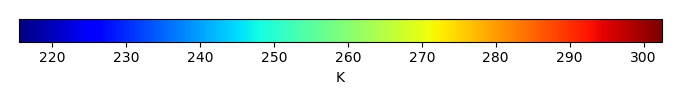MODEL MEANBIAS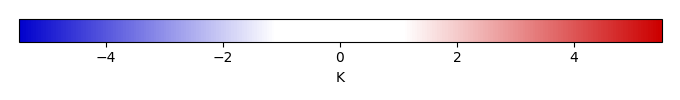BIAS SCORERMSE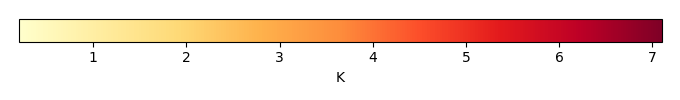RMSE SCOREBENCHMARK INTERANNUAL VARIABILITY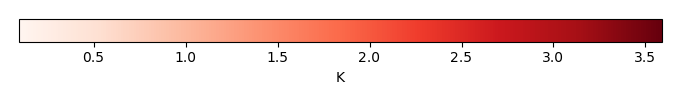MODEL INTERANNUAL VARIABILITYINTERANNUAL VARIABILITY SCOREBENCHMARK MAX MONTHMODEL MAX MONTHDIFFERENCE IN MAX MONTH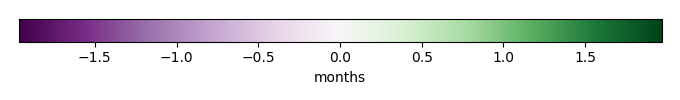SEASONAL CYCLE SCORESPATIAL TAYLOR DIAGRAMMODEL COLORS# Spatially integrated regional mean

MODEL COLORSREGIONAL MEANANNUAL CYCLEMONTHLY ANOMALYANNUAL CYCLE# All Models

BenchmarkCRUNCEPv7GSWP3v1WATCH# Data Information

creation_date: Fri Nov 1 11:52:30 PDT 2013

source_file: This product is generated from monthly CRU TS3.21 Mean Temperature observations

title: derived CRU high resolution (0.5x0.5) Mean Temperature

Approach: I simply read the monthly surface air temperature from original file, and separated the time series by a month and saved them by each month and each year.

Temporal resolution: monthly

General information: This product was derived from CRU v3.21 product.

Spatial resolution: 0.5x0.5 degree